# Quantitative Aptitude Quiz For ECGC PO 2021- 11th January

Directions (1-5): In the following questions there are two equations given. You have to solve both the equations and give answer:
(a) if x > y
(b) if x < y
(c) if x ≥ y
(d) if x ≤ y
(e) if x = y or relation between x and y cannot be established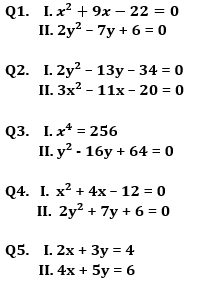Directions (6-10): Two equations I and II are given below in each question. You have to solve these equations and give answer accordingly.
(a) if x<y
(b) if x>y
(c) if x≤y
(d) if x≥y
(e) if x=y or no relation can be established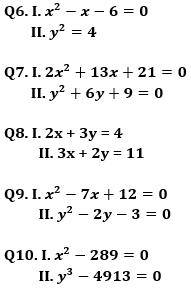Directions (11 – 15): In the following questions, two equations, I and II are given. You have to solve both the equations and give answer accordingly.
(a) if x>y
(b) if x≥y
(c) if x<y
(d) if x≤y
(e) if x=y or no relation.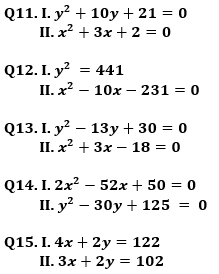Practice More Questions of Quantitative Aptitude for Competitive Exams:

###### ECGC PO Study Plan 2021

Solutions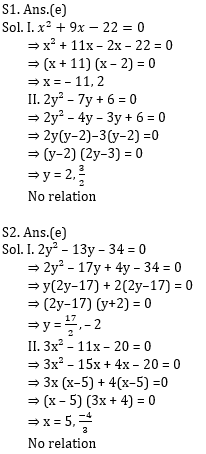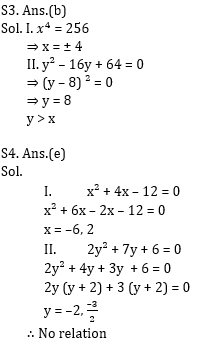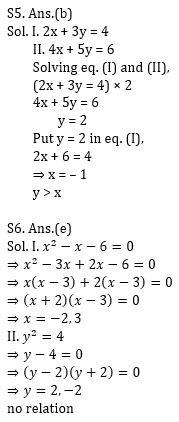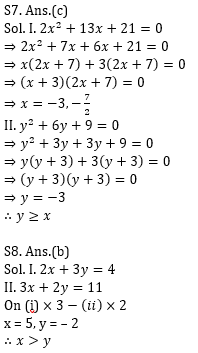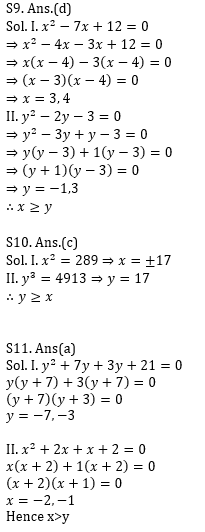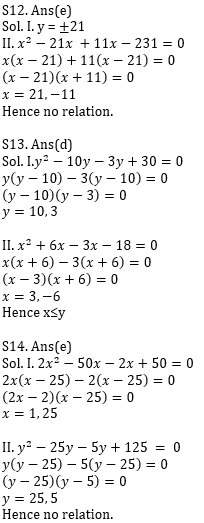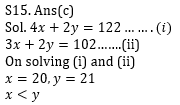Practice with Crash Course and Online Test Series for ECGC PO 2021: